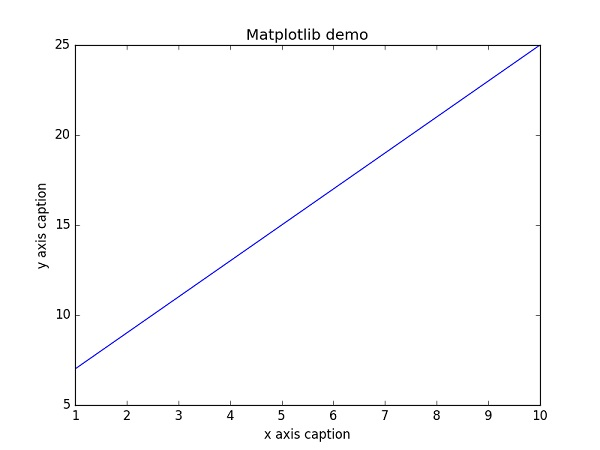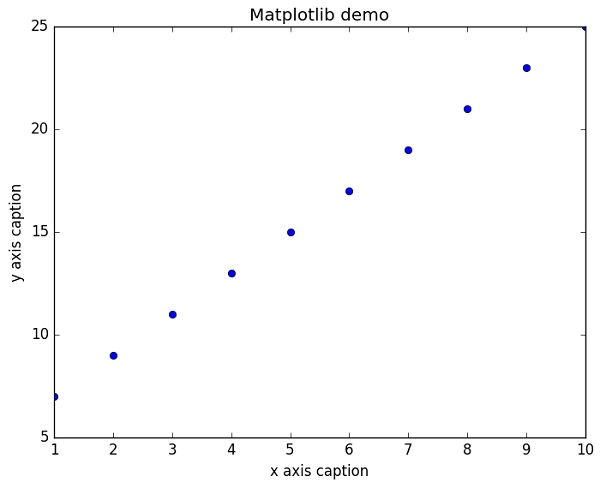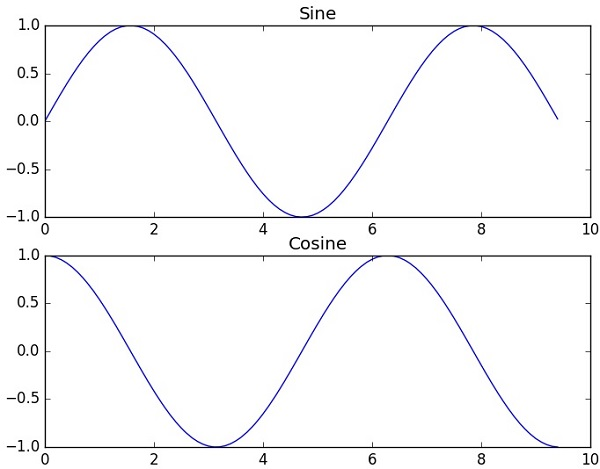# NumPy - Matplotlib

Matplotlib 是 Python 的绘图库。 它可与 NumPy 一起使用，提供了一种有效的 MatLab 开源替代方案。 它也可以和图形工具包一起使用，如 PyQt 和 wxPython。

Matplotlib 模块最初是由 John D. Hunter 编写的。 自 2012 年以来，Michael Droettboom 是主要开发者。 目前，Matplotlib 1.5.1 是可用的稳定版本。 该软件包可以二进制分发，其源代码形式在 www.matplotlib.org 上提供。

``````from matplotlib import pyplot as plt
``````

### 示例

``````import numpy as np
from matplotlib import pyplot as plt

x = np.arange(1,11)
y =  2  * x +  5
plt.title("Matplotlib demo")
plt.xlabel("x axis caption")
plt.ylabel("y axis caption")
plt.plot(x,y) plt.show()
``````

`ndarray`对象`x``np.arange()`函数创建为`x`轴上的值。`y`轴上的对应值存储在另一个数组对象`y`中。 这些值使用`matplotlib`软件包的`pyplot`子模块的`plot()`函数绘制。`'-'` 实线样式
`'--'` 短横线样式
`'-.'` 点划线样式
`':'` 虚线样式
`'.'` 点标记
`','` 像素标记
`'o'` 圆标记
`'v'` 倒三角标记
`'^'` 正三角标记
`'&lt;'` 左三角标记
`'&gt;'` 右三角标记
`'1'` 下箭头标记
`'2'` 上箭头标记
`'3'` 左箭头标记
`'4'` 右箭头标记
`'s'` 正方形标记
`'p'` 五边形标记
`'*'` 星形标记
`'h'` 六边形标记 1
`'H'` 六边形标记 2
`'+'` 加号标记
`'x'` X 标记
`'D'` 菱形标记
`'d'` 窄菱形标记
`'&#124;'` 竖直线标记
`'_'` 水平线标记

`'b'` 蓝色
`'g'` 绿色
`'r'` 红色
`'c'` 青色
`'m'` 品红色
`'y'` 黄色
`'k'` 黑色
`'w'` 白色

### 示例

``````import numpy as np
from matplotlib import pyplot as plt

x = np.arange(1,11)
y =  2  * x +  5
plt.title("Matplotlib demo")
plt.xlabel("x axis caption")
plt.ylabel("y axis caption")
plt.plot(x,y,"ob")
plt.show()
``````## 绘制正弦波

### 示例

``````import numpy as np
import matplotlib.pyplot as plt
# 计算正弦曲线上点的 x 和 y 坐标
x = np.arange(0,  3  * np.pi,  0.1)
y = np.sin(x)
plt.title("sine wave form")
# 使用 matplotlib 来绘制点
plt.plot(x, y)
plt.show()
``````## `subplot()`

`subplot()`函数允许你在同一图中绘制不同的东西。 在下面的脚本中，绘制正弦余弦值。

### 示例

``````import numpy as np
import matplotlib.pyplot as plt
# 计算正弦和余弦曲线上的点的 x 和 y 坐标
x = np.arange(0,  3  * np.pi,  0.1)
y_sin = np.sin(x)
y_cos = np.cos(x)
# 建立 subplot 网格，高为 2，宽为 1
# 激活第一个 subplot
plt.subplot(2,  1,  1)
# 绘制第一个图像
plt.plot(x, y_sin)
plt.title('Sine')
# 将第二个 subplot 激活，并绘制第二个图像
plt.subplot(2,  1,  2)
plt.plot(x, y_cos)
plt.title('Cosine')
# 展示图像
plt.show()
``````## `bar()`

`pyplot`子模块提供`bar()`函数来生成条形图。 以下示例生成两组`x``y`数组的条形图。

### 示例

``````from matplotlib import pyplot as plt
x =  [5,8,10]
y =  [12,16,6]
x2 =  [6,9,11]
y2 =  [6,15,7]
plt.bar(x, y, align =  'center')
plt.bar(x2, y2, color =  'g', align =  'center')
plt.title('Bar graph')
plt.ylabel('Y axis')
plt.xlabel('X axis')
plt.show()
``````